Select Page

# CBSE Answers for MCQ 12 Science Maths Application of Derivatives in Hindi

CBSE Answers for MCQ 12 Science Maths Application of Derivatives in Hindi to enable students to get Answers in a narrative video format for the specific question.

Expert Teacher provides CBSE Answers for MCQ 12 Science Maths Application of Derivatives through Video Answers in Hindi language. This video solution will be useful for students to understand how to write an answer in exam in order to score more marks. This teacher uses a narrative style for a question from Application of Derivatives not only to explain the proper method of answering question, but deriving right answer too.

Please find the question below and view the Answer in a narrative video format.

Question:

## Similar Questions from CBSE, 12th Science, Maths, Application of Derivatives

Question 1 : Which of the following functions are strictly decreasing on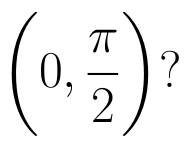(View Answer Video)

Question 2 : The rate of change of the area of a circle with respect to its radius r at r = 6 cm is : (View Answer Video)

Question 3 : The normal at the point (1, 1) on the curve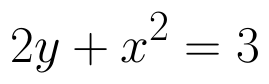is: (View Answer Video)

Question 4 : The slope of the normal to the curve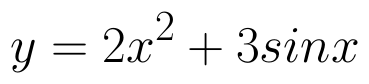at x = 0 is :

Question 5 : Find two numbers whose sum is 24 and whose product is as large as possible. (View Answer Video)

### Inverse Trigonometric Functions

Question 1 :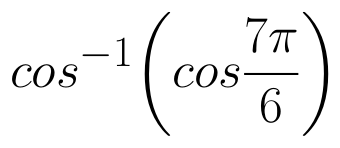is equal to :
Question 1 :  Find the second order derivative of the function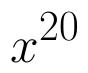. (View Answer Video)
Question 3 :  Find the second order derivative of the function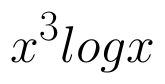. (View Answer Video)
Question 4 :  Find the second order derivative of the function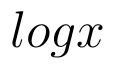. (View Answer Video)
Question 5 : Differentiate the function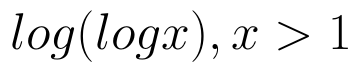with respect to x. (View Answer Video)